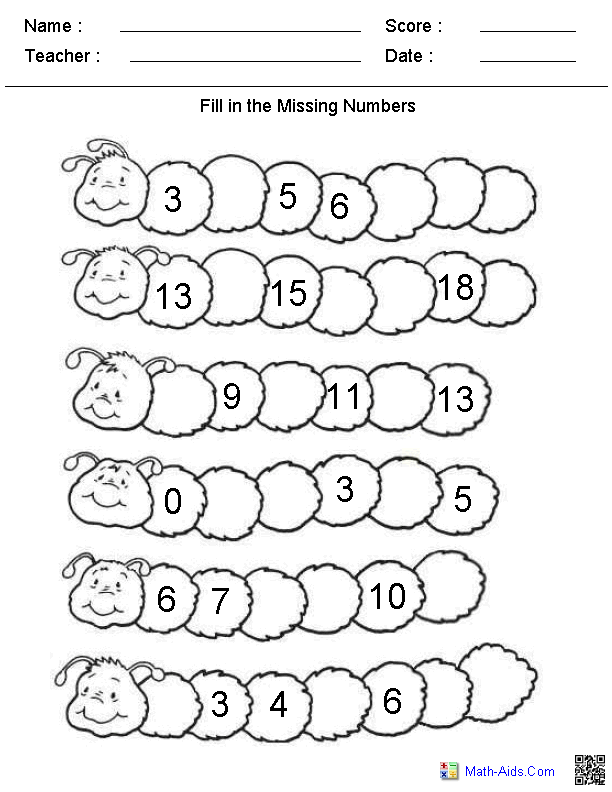lbartman.com - the pro math teacher

• Subtraction
• Multiplication
• Division
• Decimal
• Time
• Line Number
• Fractions
• Math Word Problem
• Kindergarten
• a + b + c

a - b - c

a x b x c

a : b : c

Math Worksheet Kindergarten

Public on 07 Oct, 2016 by Cyun Leekindergarten worksheets dynamically created kindergarten worksheets

Name : __________________

Seat Num. : __________________

Date : __________________

HOW MANY STARS EACH LINE ?

......
......
......
......
......
show printable version !!!hide the show

RELATED POST

Not Available

POPULAR

halloween math printable worksheets

proper fraction worksheets

worksheets for adding and subtracting fractions

printable math worksheets for kindergarten addition and subtraction

subtraction of integers worksheet

kindergarten main idea worksheets